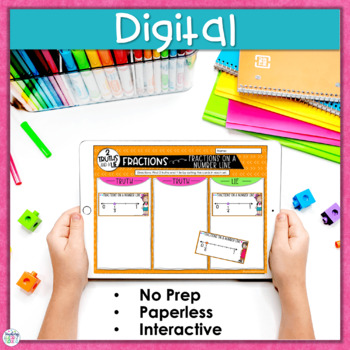# Fractions Activity | Fractions Math Center | Error Analysis | 2 Truths2nd - 4th
Subjects
Standards
Resource Type
Formats Included
• Zip
•Google Apps™
Pages
20 pagesThe Teacher-Author indicated this resource includes assets from Google Workspace (e.g. docs, slides, etc.).

### Description

Your students will love this fun and engaging hands-on fractions activity that helps them practice fractions strategies such as equivalent fractions, unit fractions, and fractions on a number line through error analysis! This QUICK PRINT AND GO or DIGITAL activity also gives students important practice in all aspects of fractions.

How to Use this Fractions Activity:

Students are given 3 fractions representations (example: equivalent fractions, unit fractions, fractions on a number lines) and must determine which 2 are true and which one is a lie.

⭐ There are 5 different activities in this resource that help students practice with equivalent fractions, unit fractions, fractions on a number line, fractions greater than one, and comparing fractions.

⭐ This 2 Truths and a Lie Fractions Error Analysis activity has so much flexibility in a printer friendly black and white version or color printables, and a digital version in Google™ Slides. Use it whichever way is best for YOU and your STUDENTS:

★ Cut and paste math centers activity

★ Print and laminate the pieces and use multiple times

★ Assign the digital version in a computer center.

→→→ PLEASE SEE PREVIEW FOR A CLOSER LOOK!

───────────────────────────

What's Included in this Resource:

Two Truths and a Lie Organizer Sheet, Fraction Representation Cards, and Answer Key for:

• Equivalent Fractions
• Unit Fractions
• Fractions on a Number Line
• Fractions Greater Than One
• Comparing Fractions

───────────────────────────

2 Truths and a Lie Fractions Activities can easily be used in these settings:

• Whole Group Instruction
• Small Group Instruction
• Centers
• Formative Assessment
• Partner Work
• Bell Work

───────────────────────────
Common Core Math Standards Addressed in this Resource:
3.NF.A.1 - Understand a fraction 1/bas the quantity formed by 1 part when a whole is partitioned intobequal parts; understand a fractiona/bas the quantity formed byaparts of size 1/b.
3.NF.A2 -Understand a fraction as a number on the number line; represent fractions on a number line diagram.
3.NF.A3 - Explain equivalence of fractions in special cases, and compare fractions by reasoning about their size.

───────────────────────────
If you and your students love this fun and engaging error analysis activity for fractions, you will love these too!

Need more engaging and rigorous fractions resources?

♥ CLICK HERE TO FOLLOW MY STORE FOR New Products, Updates, and Great Deals! ♥

"Don't forget to leave feedback -you will receive TPT credits that you can use toward future purchases!

Thank you for visiting Teaching in the Heart of Florida!

Total Pages
20 pages
Included
Teaching Duration
Other
Report this resource to TpT
Reported resources will be reviewed by our team. Report this resource to let us know if this resource violates TpT’s content guidelines.

### Standards

to see state-specific standards (only available in the US).
Understand a fraction 1/𝘣 as the quantity formed by 1 part when a whole is partitioned into 𝘣 equal parts; understand a fraction 𝘢/𝑏 as the quantity formed by 𝘢 parts of size 1/𝘣.
Understand a fraction as a number on the number line; represent fractions on a number line diagram.
Represent a fraction 1/𝘣 on a number line diagram by defining the interval from 0 to 1 as the whole and partitioning it into 𝘣 equal parts. Recognize that each part has size 1/𝘣 and that the endpoint of the part based at 0 locates the number 1/𝘣 on the number line.
Explain equivalence of fractions in special cases, and compare fractions by reasoning about their size.
Understand two fractions as equivalent (equal) if they are the same size, or the same point on a number line.

### Questions & Answers

Teachers Pay Teachers is an online marketplace where teachers buy and sell original educational materials.